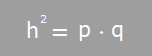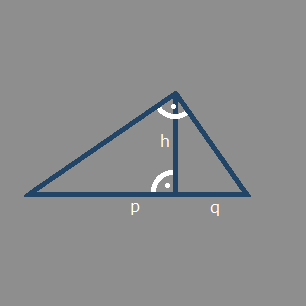What is the Pythagorean Theorem?

The Theorem applies to right triangles only.

Given are the partial lengths of the hypotenuse that are constructed with an orthogonal line (rotation by 90 degrees) which goes through the point that lies to the opposite of the hypotenuse. With this information you are able to calcualte the height. Here is the formula:You may click on the formula to see other evaluations of the equation.

What will this site do for you?

You will be able to calculate height and the partial lengths of the hypothenuse of right triangles with the Geometric Mean Theorem.

You may enter positive floating point numbers. Any other input is considered as missing. No evaluation will take place then.

Due to the Geometric Mean Theorem you have to provide at least 2 elements to calculate the third one.

After you entered 2 elements of the equation click on "Calculate".

If you have a problem to match the triangle shape to correctly assign the sides you may click on the triangle to rotate it in 90 degrees.
Geometric Mean Theorem

Calculates the height of a triangle and partial lengths of the hypotenusep q h